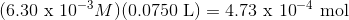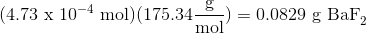# College Chemistry : Solubility Product Constant

## Example Questions

### Example Question #1 : Solubility Product Constant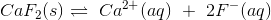Express the solubility product constant expression for the given reaction.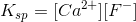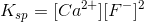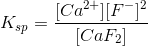Explanation:

The equilibrium given tells us how the solid dissolves in solution:However, the solubility product constant (Ksp) given tells us the degree by which a solid dissolves in solution. The larger the Ksp, the more soluble a substance is in water. Writing this expression follows the same rules as other equilibrium constant expressions. Therefore solids and water (when it is the solvent) are omitted from this expression. You must raise the concentration of the substances involved to the power of its coefficient.

For the chemical reaction given, the Ksp is:### Example Question #2 : Solubility Product Constant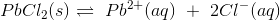Express the solubility product constant expression for the given reaction.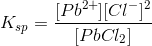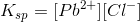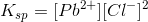Explanation:

The equilibrium given tells us how the solid dissolves in solution:However, the solubility product constant (Ksp) given tells us the degree by which a solid dissolves in solution. The larger the Ksp, the more soluble a substance is in water. Writing this expression follows the same rules as other equilibrium constant expressions. Therefore solids and water (when it is the solvent) are omitted from this expression. You must raise the concentration of the substances involved to the power of its coefficient.

For the chemical reaction given, the Ksp is:### Example Question #3 : Solubility Product Constant

How many grams of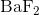are dissolved in 75.0 mL of a saturated solution of?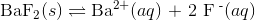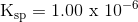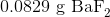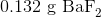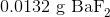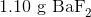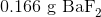Explanation:

To calculate how many grams ofare dissolved in the solution, we first solve for the molarity of the solution.

Using the dissociation equationWe can write out the equation for the solubility product constant as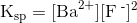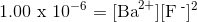Because there are 2 fluoride ions for every barium ion, we can rewrite the equation as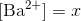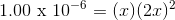Now solve for x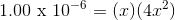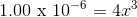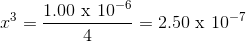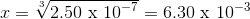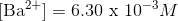Solve for the concentration of dissolved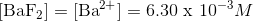Now that we have the concentration of dissolved, we can calculate how many grams are dissolved in the solution